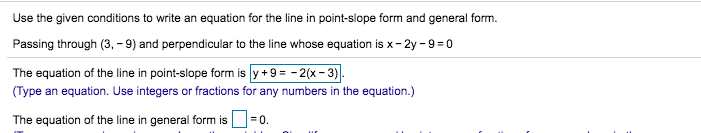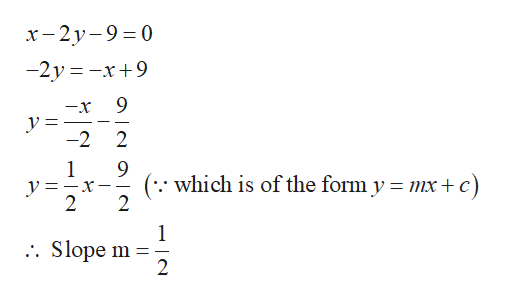# Use the given conditions to write an equation for the line in point-slope form and general form.Passing through (3, -9) and perpendicular to the line whose equation is x-2y -9 0The equation of the line in point-slope form is y +9=-2(x-3).(Type an equation. Use integers or fractions for any numbers in the equation.)The equation of the line in general form is0.

Question
100 views

Did I do this correctly? and How would I solve for the general form?help_outlineImage TranscriptioncloseUse the given conditions to write an equation for the line in point-slope form and general form. Passing through (3, -9) and perpendicular to the line whose equation is x-2y -9 0 The equation of the line in point-slope form is y +9=-2(x-3). (Type an equation. Use integers or fractions for any numbers in the equation.) The equation of the line in general form is 0. fullscreen
check_circle

Step 1

The line passes through (3,–9) and perpendicular to the equation x-2y-9=0.

Determine the slope m o...help_outlineImage Transcriptioncloseх- 2у-9%3D0 -2y x+9 9 -x -2 2 1 which is of the form y mx + 2 c) 2 1 . Slope m 2 fullscreen

### Want to see the full answer?

See Solution

#### Want to see this answer and more?

Solutions are written by subject experts who are available 24/7. Questions are typically answered within 1 hour.*

See Solution
*Response times may vary by subject and question.
Tagged in

### Algebra